## RD Sharma Solutions for Class 6 Chapter 15 Pair of Lines and Transversal Free Online

Exercise 15.1 page: 15.2
1. Identify parallel line segments shown in Fig. 15.6.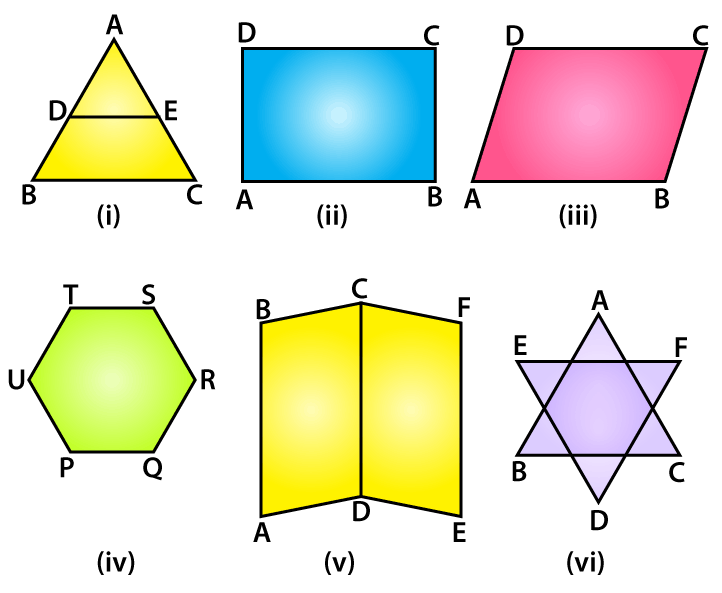Solution:
(i) From the figure we know that BC || DE.
(ii) From the figure we know that AB || DC, AD || BC.
(iii) From the figure we know that AB || DC and AD || BC.
(iv) From the figure we know that PQ || TS, UT || QR and UP || SR.
(v) From the figure we know that AB || EF || CD, BC || AD and CF || DE.
(vi) From the figure we know that EF || BC, AB || DF and AC || DE.
2. Name the pairs of all possible parallel edges of the pencil box whose figure is shown in Fig. 15.7.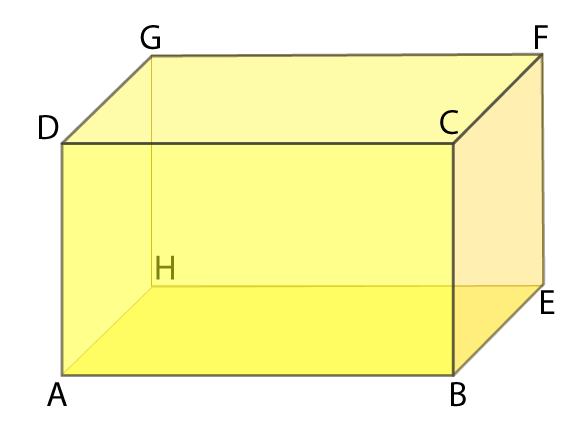Solution:
The pairs of all possible parallel edges of the pencil box are
AB || DC || HE || GF and AD || GH || BC || EF
3. In Fig. 15.8, do the segments AB and CD intersect? Are they parallel? Give reasons.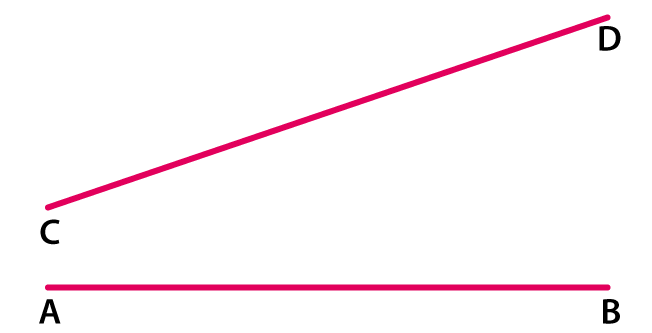Solution:
No, AB and CD do not intersect but they can intersect if extended further. No AB and CD are not parallel since, the distance between them is not constant.
4. State which of the following statements are true (T) or which are false (F):
(i) If two lines in the same plane do not intersect, then they must be parallel.
(ii) Distance between two parallel lines is not same everywhere.
(iii) If m ⊥ l, n ⊥ l and m ≠ n, then m || n.
(iv) Two non-intersecting coplanar rays are parallel.
(v) If ray AB || line m, then line segment AB.
(vi) If line AB || line m, then line segment AB || m.
(vii) No two parallel line segments intersect.
(viii) Every pair of lines is a pair of coplanar lines.
(ix) Two lines perpendicular to the same line are parallel.
(x) A line perpendicular to one of two parallel lines is perpendicular to the other.
Solution:
(i) True
(ii) False
(iii) True
(iv) False
(v) True
(vi) True
(vii) True
(viii) False
(ix) True
(x) True

Exercise 15.2 page: 15.6
1. In Fig. 15.17, line n is a transversal to lines l and m. Identify the following:
(i) Alternate and corresponding angles in Fig. 15.17 (i).
(ii) Angles alternate to ∠d and ∠g and angles corresponding to ∠f and ∠h in Fig. 15.17 (ii).
(iii) Angle alternative to ∠PQR, angle corresponding to ∠RQF and angle alternate to ∠PQE in Fig. 15.17 (iii).
(iv) Pairs of interior and exterior angles on the same side of the transversal in Fig. 15.17 (ii).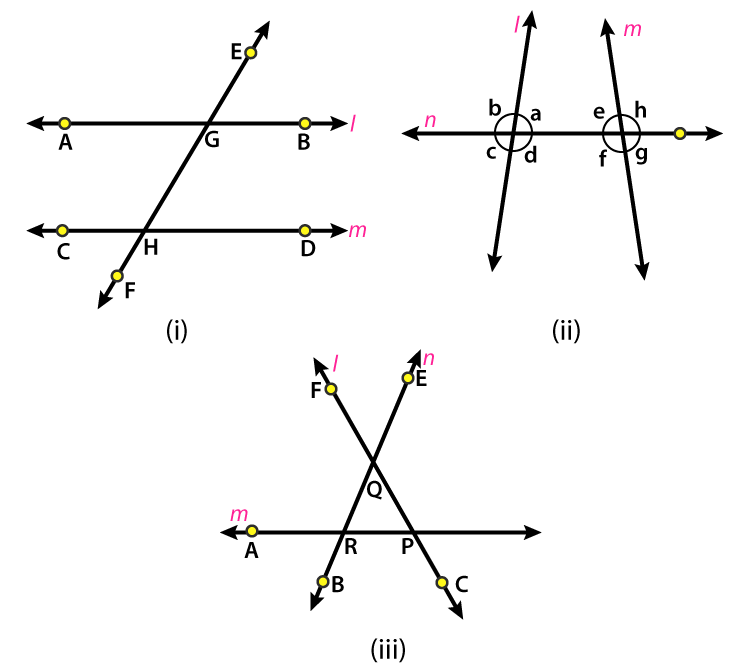Solution:
(i) Alternate interior angles are ∠BGH and ∠CHG; ∠AGH and ∠CHF
Alternate exterior angles are ∠AGE and ∠DHF; ∠EGB and ∠CHF
Corresponding angles are ∠EGB and ∠GHD; ∠EGA and ∠GHC; ∠BGH and ∠DHF; ∠AGF and ∠CHF.
(ii) Angles alternate to ∠d and ∠g are ∠e and ∠b and angles corresponding to ∠f and ∠h are ∠c and ∠a.
(iii) From the figure we know that l is transversal to m and n.
Angle alternate to ∠PQR is ∠QRA
Angle corresponding to ∠RQF is ∠BRA
Angle alternate to ∠PQE is ∠BRA
(iv) Interior angles are ∠d, ∠f and ∠a, ∠e and exterior angles are ∠c, ∠g and ∠b, ∠h
2. Match column A and column B with the help of the Fig. 15.18:
Column A Column B
(i) Vertically opposite angles (i) ∠PAB and ∠ABS
(ii) Alternate angles (ii) ∠PAB and ∠RBY
(iii) Corresponding angles (iii) ∠PAB and ∠XAQ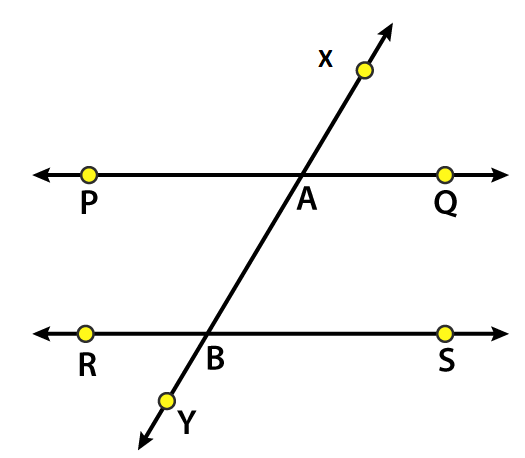Solution:
(i) ∠PAB and ∠XAQ are vertically opposite angles
(ii) ∠PAB and ∠ABS are alternate angles
(iii) ∠PAB and ∠RBY are corresponding angles
Courtesy : CBSE